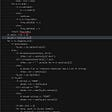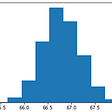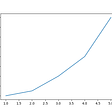# Python: Data manipulation and simulation

In this post, we’ll walk through basic techniques of data manipulation and simulation with Python.

Data manipulation

A. Group aggregation

Given a data frame, say we want to summarize customer orders by different genders, we can use a simple groupby and agg function:

`values_gender = csv_file\                       .groupby(['gender']) \                       .agg(avg_order_values=('value','mean'),\                            count_order=('value', 'size')) \                       .reset_index()`

In the code, we create a new data frame, values_gender, from the original data, csv_file. Groupby specifies which variable to summarize at, similar to “group by” in SQL. Agg let you specify which variable to be summarized at Gender level, and which summary statistics to use. In this example, we create a new column, avg_order_values, as the mean of order values per gender; we also create a column, count_order, as the number of orders per gender. The last line, reset_index(), will ensure Gender is not defined as an index in the new data frame. I used backslash to tell Python the next line belongs to the same command.

B. Lambda functions

Lambda functions allow us to write simple short functions, usually used with Apply functions. For example, say we want to replace gender representations from “male” and “female” to “M” and “F.” We go with if conditions:

`values_gender['gender'] = values_gender['gender']\                          .apply(lambda x:\                          "F" if x=="Female" else "M")`

In the above example, x is the Gender column in the data frame Values_Gender. We tell Python to replace “Female” with “F” in the Gender column (x); and replace anything not “Female” with “M.”

C. Merge (equivalent to “Join” in SQL)

The Python syntax of merge statements is similar to SQL syntax of join statements. For example, say we want to merge the created Avg_Order_Values and Count_Order columns from Section A with the original csv_file data frame:

`csv_file.merge(\        values_gender[['gender','avg_order_values','count_order']],\              left_on='gender', right_on='gender', how = 'left')\               [['datetime','gender']]`

In the above merge statement, we add Average_Order_Values and Count_Order columns from the data frame Values_Gender to the data frame csv_file. In this case, all the “Male” genders will have the same Average_Order_Values and Count_Order, as we use a left join (in the “how” clause) and retain all the rows in the left datasets, csv_file. We can rewrite the same code in SQL as below:

`SELECT datetime, gender FROM csv_file aLEFT JOIN (SELECT gender, avg_order_values, count_order            FROM values_gender) bON a.gender = b.gender`

For more translation between Python and SQL, see: https://medium.com/jbennetcodes/how-to-rewrite-your-sql-queries-in-pandas-and-more-149d341fc53e

Data simulation

Simulation is popular in marketing. We need basic probability theory to make assumptions and decide how we simulate data. Say we want to simulate how many orders a customer will make in lifetime.

Assume a customer’s transaction numbers follow a poisson distribution with the expected value lambda = c for some constant c. Poisson distribution is useful for modeling the uncertainty in counts. It comes with the following assumptions:

a. Each transaction is independent from each other (not sequentially related). It’s a hard assumption, as customers may purchase in groups (with coupons) or your store may be featured in local newspapers.

b. Constant lambda — average transaction per time period doesn’t change; variation of transaction also doesn’t change with time. If you use “day” as a unit time interval, the number of transactions at your store can vary by hours, as you might have more customers at dinner time. However, it’s difficult to choose a unit time, because even if you choose “month,” you still want to take seasonality effects into account.

c. Two transactions don’t occur simultaneously. This gives us the opportunity to think of each transaction as a Bernoulli trial individually. Further, Poisson approximates Binomial distribution when a sample size is infinitely large and a success probability is infinitely small. In reality, we rarely know the sample size or success probability that are required for binomial simulation; however, it’s not too difficult to get an estimate of the expected rate, lambda, from the number of transactions we get from a unit period.

Taking lambda = 4, we simulate:

`from scipy.stats import poissoncsv_file['predicted_transactions'] = np.random.poisson(4)`

https://towardsdatascience.com/poisson-distribution-intuition-and-derivation-1059aeab90d

In reality, we might not want to make a solid guess of the value of lambda in Poisson. To simulate lambda, we can use gamma distribution, where the random variable is always positive and right skewed. Will talk about gamma later!

If you like my writing, please remember to send a clap!

--

--

--

## More from Analytics Vidhya

Analytics Vidhya is a community of Analytics and Data Science professionals. We are building the next-gen data science ecosystem https://www.analyticsvidhya.com

## How Much Do Data Scientists Make?## The curious case of applying nlp to Twitter## Can data tell you if your baby is hungry?## Predicting Hotel Bookings with User Search Parameters## Marketing automation — Recommendation systems## Practical Statistics with Python (1): Distributions, Theorem and Confidence Intervals## Qingchuan Lyu

Data Engineering, Causal Inference & Predictive Analysis

## Vector autoregression forecast on chemical data of The Antwerp Maritime Academy## How to make interpolated lines in Python## An Introduction To Statistics With Python — Part 1## Python Pandas Fast Forward with a Case Study